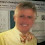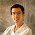## Wednesday, September 14, 2011

### Conventions in Math- just to make the rules work or more?

1. Why is
a1/2 = sqrt(a)?
true? To make the rule
ax+y=ax a y
work out.
2. Why is
(∀ x ∈ ∅)[P(x)]
true? To make the rule
(∀ x ∈ A ∪ B)[P(x)] iff (∀ x ∈ A)[P(x)] AND (∀ x ∈ B)[P(x)]
work out.
3. Why is the sum over an empty index set equal to 0 and the product over an empty index set equal to 1? Same reason- it makes various math laws work out.
For the second and third item on the above list I would say its not JUST to make some rule work out, it also makes sense. But the first item, that a1/2 = sqrt(a), seems to only make sense in terms of making a rule work out and not for any other reason. I have no objection to the rule; however, if you have a REASON other than it makes the rules work for this convention, please leave a comment.

1.Because a^{1/2} = exp( {1/2} ln a)

One could argue that this is also 'just making a rule work out', but I'd say that the theory of the complex exponential and natural logarithm is natural and rich enough to give serious weight to the argument that it 'makes sense'.

2.My favorite of these is 0^0, which is defined to be 1, but there are some semi-plausible sounding reasons you might be compelled to define it to be 0, or even leave it undefined. (Of course, if you actually did something rash like that, then it would no longer be true that for every real x, e^x = sum_{i=0}^{infty} x^i/i!)

3.Why is a^2 = a*a? Is this equivalent to square-root question or more fundamental?

4.(1) holds because sqrt{a} is a shortcut for a^{1/2}.

(2) holds because "(∀ x ∈ ∅)[P(x)]" is a shortcut for "∀ x ((x ∈ ∅) => P(x))". Since "(x ∈ ∅)" is always false, "((x ∈ ∅) => P(x))" is always true.

(3) In any group, the product of elements in the empty set equals the identity element of the group. Now 0 is the identity element of the additive group, and 1 is the identity element of the multiplicative group.

5.I would say that for n a natural number \ge 1

a^n = a * a * ... * a (n times)
is the DEFINITION of a-to-a-power.

All the rest of the rules (e.g., a^{-1} = 1/a
and a^{1/2}=sqrt(a), etc) are made up in order to make
the addition rule WORK. So I think that a*a=a^2 is
quite diff from a^{1/2} = sqrt(a).

Again- I AGREE with the definitions but wonder if there
is an alternative explanation of them.
I was thinking of a SIMPLE explanation, so
Richard Elwes comment, while very interesting, is not
quite what I am looking for.

6.In third grade I was taught the following regrettable yet unforgettable mnemonic:

--------------
"Minus times minus equals plus;
the reason for this, we will not discuss."
--------------

A graduate-level echo of this is found in William L. Burke's samizdat masterpiece Div, Grad, Curl are Dead:

-----------------
Mathematician: When do you guys treat dual spaces?

Scientist/Engineer: We don't.

Mathematician: What! How can that be?
-----------------

Burke goes on to say:

-----------------
"You may have taken a course on linear algebra. The purpose of this book is to repair the omissions of such a course, which now is typically only a course on matrix manipulation."
-----------------

Here Burke's point is that mathematical ideals of naturality and universality (which nowadays are ubiquitous in the mathematics curriculum and which I take to be the broad theme of GASARCH's post) are making their way only slowly and painfully into the science-and-engineering curriculum.

It is not at all clear (to me) how to recognize "naturality and universality" in complexity theory, and yet definitely I wish for this ability; remarks (from anyone) in this regard would be very welcome. And if someone (not me!) has the requisite chutzpah to post it as a question/community wiki on TCS StackExchange, that might be fun too.

7.As pointed out by Thurston, any fundamental mathematical concept worth its salt (and exponentiation is certainly one of these) should have a number of different interpretations, and the one that one is first exposed to (in this case, iterated multiplication) is not necessarily the "best" one for generalisation. One already sees this with, say, multiplication; how does one justify (-2)*(-3) = 6 using the iterated addition interpretation of multiplication?

I discuss different interpretations of exponentiation, by the way, at

Iterated multiplication is interpretation 4. While this interpretation does not cover a^{1/2}, other interpretations (in my list, 5, 6, 7, and 9) do. ("Making the rules work" is interpretation 6.)

8.I agree with what previous commenters have said: a^{1/2} is *** by definition *** the number that when squared gives a. So it's not "to make the rules work out", it's just a definition (that, fortunately, happens to be consistent with the rules).

9.Daina Taimina's book Crocheting Adventures with Hyperbolic Planes, which won last year's coveted Diagram Prize, includes a Foreword by Bill Thurston that expounds upon the theme of Terry Tao's post (above) as follows:

-----------------------
Mathematics is an art of human understanding … Our brains are complicated devices, with many specialized modules working behind the scenes to give us an integrated understanding of the world. Mathematical concepts are abstract, so it ends up that there are many different ways that they can sit in our brains.

A given mathematical concept might be primarily a symbolic equation, a picture, a rhythmic pattern, a short movie---or best of all, an integrated combination of several different representations. These non-symbolic mental models for mathematical concepts are extremely important, but unfortunately, many of them are hard to share.

Mathematics sings when we feel it in our whole brain. People are generally inhibited about even trying to share their personal mental models. People like music, but they are afraid to sing. You only learn to sing by singing.
-----------------------

I would like to share one idiom that engineers commonly employ in choosing among mathematical conventions. The idiom draws upon ideas that are presented in three highly-rate MathOverflow (MOF) posts: (1) Gil Kalai's MOF Wiki Fundamental examples, (2) Tao's comment upon the MOF Wiki In what ways is physical intuition about mathematical objects non-rigorous?, and (3) Terry Tao's comment upon the MOF Wiki Still difficult after all these years.

The basic idiom is to take any fundamental example (per Kalai) that is associated to a set of mathematical conventions (per Gasarch) and run that example backwards. If something physically or informatically useful happens (per Tao's first MOF post), good! Otherwise, adjust the conventions/definitions (per Tao's second MOF post) until something good does happen.

The practical point of this engineering idiom is summarized in an old joke: "Q: What happens when you play a country-and-western song backwards? A: You get out of jail, sober up, find a job, fix your truck, then your spouse comes back to you and your dog does too."

For example, the strategy "Play it again, … this time backwards" is very natural in complexity theory, in which algorithms "played backwards" lead naturally to the study of trapdoor functions. But obstructions are encountered too. In particular, generic examples of algorithms in P are infeasible to construct, because so many of their key properties are undecidable (per Hartmanis). That is why we engineers would be happy to see the conventional definitions of P adjusted to remove this obstruction.

Quantum computers too (error-correcting ones especially) perform a natural and useful function when they are "played backwards", namely they operate as engines for quantum separative transport. And the low-entropy quantity they distill, namely quantum coherence, is transformationally valuable for purposes of both sensing and computation.

That bottom line is that (for engineers) good mathematical definitions and conventions help us create novel systems, take them apart, and run them backwards, all to useful purpose.

10.1)
a^1/2 = sqrt{a} isn’t made true in order to make a^(x+y) = a^x*a^y true.
a^1/2 = sqrt{a} is a consequence of the rule a^(x+y) = a^x*a^y
Proof:
Every positive real number has tow roots b and –b, let’s assume sqrt{} returns the positive root.
Then by the definition of the sqrt{} function sqrt{a} is the positive number b such that b*b = a (b is unique, this can bebproven easily)
but the number a^1/2 is just like sqrt{a} because a^1/2*a^1/2 = a^(1/2 + 1/2) = a^1 = a (this step is justified by the rule a^(x+y) = a^x*a^y)
since b is unique it follows that sqrt{a} = a^1/2 if a^1/2 > 0
else sqrt{a} = -a^1/2
A comprehensive Abstract algebra text should contain proofs that show that these rules are consequences of the definition of exponentiation.
Here is a good one that contains the proofs: Abstract Algebra: A Comprehensive Treatment (Chapman & Hall/CRC Pure and Applied Mathematics) by Claudia Menini and Freddy Van Oystaeyen

2)
(∀ x ∈ ∅)[P(x)] is true because it can’t be false, remember that a true statement in logic is nothing other than a statement that can’t be false.

Proof: if (∀ x ∈ ∅)[P(x)] is false
then there must exist at least one x ∈ ∅ such that P(x) is false
but it is absurd to say that the empty set contains an element
hence the statement (∀ x ∈ ∅)[P(x)] can’t be false so it must be true
3)
In my opinion the assigning of 0 and 1 to the summation over an empty set and the product over an empty set respectively is natural choice that makes summations and products convenient. With these rules on can express the sum or products of elements in a set as the sum or products of the elements in its disjoint subsets without worrying about empty subsets.
For example: A = {1,2} = {} U {1,2}
Sum(A) = Sum({} U {1,2}) = sum({}) + sum{{1,2}} = 0 + 3 = 3.
Product(A) = Product({} U {1,2}) = Product({} *Product( {1,2}) = 1 * 2 = 2